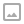This page looks best with JavaScript enabled

## Creating a Stepped Gradient Shader in Unity

·   ·  ☕ 2 min read

Let’s build a stepped gradient in Unity! This is a form of gradient with hard transitions between the different keyframes in the gradient which creates clear blocks of color like you might see on a flag or logo. To do this we’ll take advantage of the lerp and step function.

The lerp(x, y, s) function takes 3 arguments and standards for linear interpolation. This means a constant transition occurs between the left-hand side (x) and right-hand side (y) as the value (s) moves between 0 and 1. For example: lerp(0, 10, 0.5) will return 5.

The step(l, r) function takes 2 arguments and returns either 0 or 1 depending on if the left-hand side (l) is greater than the right-hand side (r). For example step(5, 1) will return 0 because 5 is greater than 1, if the arguments are reversed step(1,5) would return 1.

We can use this behavior to define our gradient. Each “key” in the gradient can be represented as a step function that either selects the new value in the gradient or returns the original values. The lerp function can provide that color selecting option by providing the color states as the left and right arguments in the lerp.

Here is an example of the 3 color gradient fragment shader we built:

// This example code is for a Unity unlit shader
fixed4 frag (v2f i) : SV_Target
{
// Create a gradient transitioning between three colors (red, green and blue).
float4 result = float4(1, 0, 0, 1); // red from 0 to 0.33
result = lerp(result, float4(0, 1, 0, 1), step(0.33, i.uv.x)); // green from 0.33 to 0.67
result = lerp(result, float4(0, 0, 1, 1), step(0.67, i.uv.x)); // blue from 0.67 to 1.0
return result;
}


Here’s an example of what this shader technique might look when applied like a flag:

You can see an example of this flag applied to the Windows Terminal (with full source code) here: https://worldofzero.com/posts/pride-windows-terminal/

I’ve explored step and smoothstep in more depth in this video: https://youtu.be/-vGNd8pnbYs
Join the World of Zero Discord Server: https://discord.gg/hU5Kq2uWRITTEN BY
Sam Wronski
Maker of things, currently helping build cloud things @ Microsoft. World of Zero is a personal project disconnected from my professional work. Lets make something awesome together!